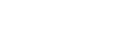## Percentage of open questions with answers, by quarter

0

(click on this box to dismiss)

Number of open questions, percentage that have at least one answer, and the percentage that have at least one *good* answer (Score > 0). Question scores are ignored.Q&A for Ubuntu users and developers

``````with quarters as (
SELECT
DATEPART(yyyy, Question.CreationDate) as Year,
DATEPART(q, Question.CreationDate) as Quarter,
COUNT(distinct Question.ID) as Total,
COUNT(DISTINCT CASE when Question.AnswerCount > 0 then Question.ID else null END) as Answered,
FROM Posts as Question
WHERE Question.PostTypeId = 1
AND Question.ClosedDate is null
AND Question.CommunityOwnedDate is null
GROUP BY DATEPART(yyyy, Question.CreationDate)
, DATEPART(q, Question.CreationDate)
)

select
Year,
Quarter,
Total as Questions,
CAST(convert(numeric(5,2), cast(Answered as float) * 100 / Total) as VARCHAR(10)) + '%' as [Answered],
CAST(convert(numeric(5,2), cast(GoodAnswer as float) * 100 / Total) as VARCHAR(10)) + '%' as [GoodAnswer]
from quarters
order by Year, Quarter``````

### Enter Parameters

Options:
-Hold tight while we fetch your results
:records returned in :time ms:cached